# Team:HokkaidoU Japan/Promoter

(Difference between revisions)
 Revision as of 08:32, 27 October 2013 (view source)Naoya (Talk | contribs)← Older edit Revision as of 09:55, 27 October 2013 (view source)Nousan (Talk | contribs) Newer edit → Line 33: Line 33:
-
Fig. 1 mRNA transcription starts with promoter engagement, continues to initiation, elongation, and then it comes to termination (omitted in the figure).
+
fig. 1 mRNA transcription starts with promoter engagement, continues to initiation, elongation, and then it comes to termination (omitted in the figure).
Line 55: Line 55:
-
Fig. 2 Consensus sequence shown in review article in 1983 .
+
fig. 2 Consensus sequence shown in review article in 1983 .
-
Fig. 3 Consensus sequence prepared in 2007 .
+
fig. 3 Consensus sequence prepared in 2007 .
Line 90: Line 90:
-
Fig. 1 Visualized data. A portion enclosed with red square is randomized -35 region.
+
fig. 1 Visualized data. A portion enclosed with red square is randomized -35 region.
-
Fig. 2 Promoters distribution. The result is an approximate normal distribution.
+
fig. 2 Promoters distribution. The result is an approximate normal distribution.

# Maestro E.coli

## Promoter# Overview

Proteins are expressed in mainly 2 steps. First mRNA is polymerized using DNA as a template. Then ribosome binds mRNA and translates it into protein.

Promoter is a DNA sequence initiating transcription from DNA to mRNA. If transcriptional efficiency is defined as "promoter strength", stronger promoter has ability to transcribe more mRNA. This should lead in stronger expression of proteins.

We have created several promoters by randomization of -35 sequence followed by selection. In promoters -35 region is responsible for supporting binding of RNA polymerase (RNAP). This interaction results in closed complex which is rate-limiting step. We focused on this rather transparent function to introduce variability in promoter strength.

We explain the importance of promoter sequence. But before that let's look how RNA binds to a promoter with the help of fig.1.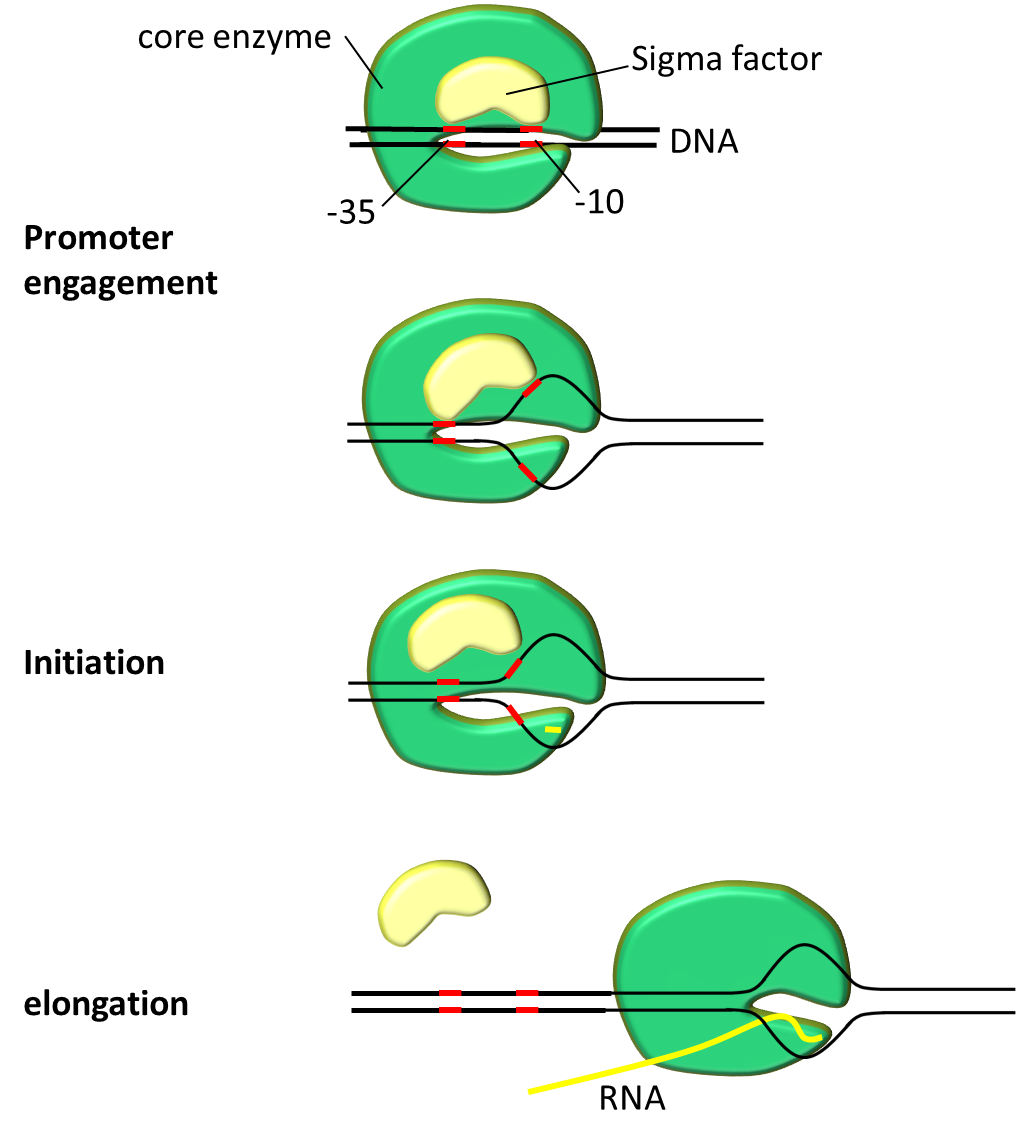fig. 1 mRNA transcription starts with promoter engagement, continues to initiation, elongation, and then it comes to termination (omitted in the figure).

First transcription complex must be formed. Transcription complex polymerizes mRNA in 2 steps. Initiation step starts polymerization followed by elongation step. Promoter serves crucial role on engagement and initiation. After closed complex formation DNA double helix pulled apart to form transcription bubble. During this closed complex changes into open complex. This marks the beginning of mRNA polymerization. Transcription bubble exposes deoxyribonucleotides to form new hydrogen bonds with ribonucleotides. In short DNA serves as template to make mRNA.

## Transcription factors related to Promtoer

RNA complex consist of 5 core enzymes and a σ factor. σ factor plays crucial role in promoter recognition. It recognizes and binds to promoter region on DNA sequence and helps to assemble the core enzyme and start transcription. σ factor has several analogs, E. coli which is widely used bacteria by iGEMers is using σ70 for house-keeping gene expression at exponential growth. Bacterial promoter can be roughly divided into three regions; -10 region, spacer and -35 region. Bases in promoter are numbered in descending order from transcription start base which is defined as +1.

-10 region
The -10 region is structurally very important because it is initiates promoter melting in RNAP-promoter complex. This is essential to form open complex. Promoter consensus sequence is TATAAT at -12 to -7 position.
Spacer
Spacer is thought to increase flexibility of σ factor binding requirements.
-35 region
-35 region is second in importance to -10. It does not energetically contribute to promoter melting. There reports on promoters without -35 region. In those case TG motif at about -16 is thought as alternative. -35 consensus sequence is TTGACA at from -36 to -31.

Promoters function to bind RNAP is a reason it is genetically well preserved. Most frequently conserved residues in the sequence make a "consensus sequence". In 1983, -35 and -10 consensus was showed to be TTGACA and TATAAT respectively [Fig 2]. Horizontal axis of the figures represents the position upstream of translation ignition point. Letter at the top of the figure signifies more than over 39% occurrence of that letter at that position. Larger occurrence over 54% is represented as upper case letter. Consensus sequence published by Marjan De Mey et al. (2007) shows that -10 and -35 region is highly preserved [Fig 3]. There other less preserved regions. The tetramer (TRTG) upstream from -10 region is called TG motif. Upstream of -35 region is UP element and downstream of -10 region is discriminator region. These sequences are thought to bind core enzymes. So these sequences are also well conserved. Each sequence is important to control promoter strength.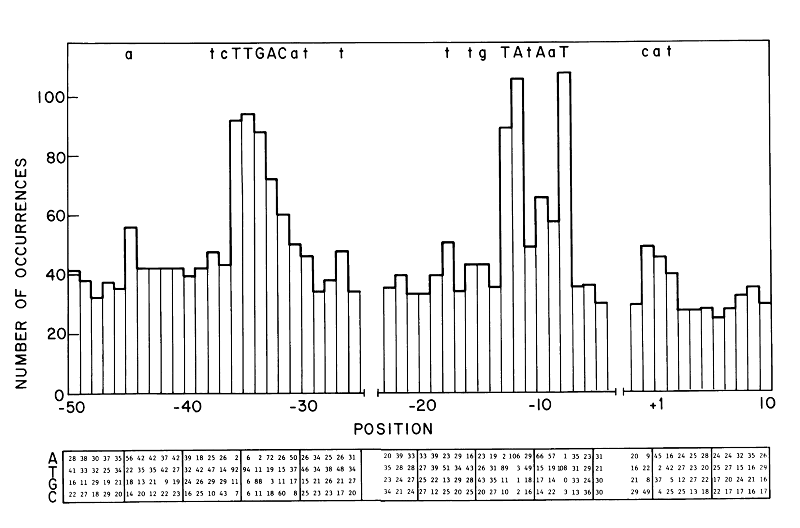fig. 2 Consensus sequence shown in review article in 1983 .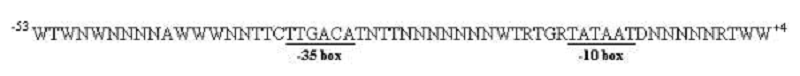fig. 3 Consensus sequence prepared in 2007 .

So we went and designed "consensus promoter". It should have strongest binding energy to RNAP. By adding mutations to -35 we sought to construct promoters with various binding energies. There are three reasons why we used -35 region.

First, -35 region is just supporting binding with σ factor. It has less vital role compared to -10 region, which energetically contributes to formation of open complex. Having this in mind we changed -35 region to easily change promoter binding strength without severe errors in promoter function.

Second, RNAP and promoter binding orchestrated by σ factor binding. Complex formation is thought to be rate-limited step. We thought that -35 region performs a simpler function. For this reason, mutations at -35 region can be thought as more structurally transparent.

Recently published research reported the making of promoter family by randomizing both -35 and -10 regions, changing spacer length. However it would be too much of the task for us to make some many changes. By changing hexamer sequence of -35 region there are 4096 variation. This number is a lot smaller compared to mutating every promoter position. So we can get result with a smaller library size.

With these 3 reasons we went on to construct our promoter family.

# MODELING

We tried to theoretically predict the strength distribution of 4096 promoters, which were artificially created by random mutation. We followed these 3 steps, referring the study by Brewster et al..

1. Calculate the binding energy of each promoter and σ-factor using the sequence
2. Convert the binding energy to the probability that RNAP binds promoter using the method of statistical mechanics
3. Utilizing the binding probability as the transcription efficiency

## STEP 1: Calculation of Binding Energy

First, we found the binding energy of RNAP and our promoters. As we mutated only -35 region, we only use this region for calculations. Here we define the binding energy ε as the energy released by RNAP’s binding to promoter. Simply saying, the higher is the binding energy, the stronger is the binding. We referred the data in Kenney et al. to calculate each binding energy.

The distribution of computed 4096 promoters' binding energies is shown below. The horizontal axis stands for ε (at 0.05 kT intervals) and the vertical axis sample number.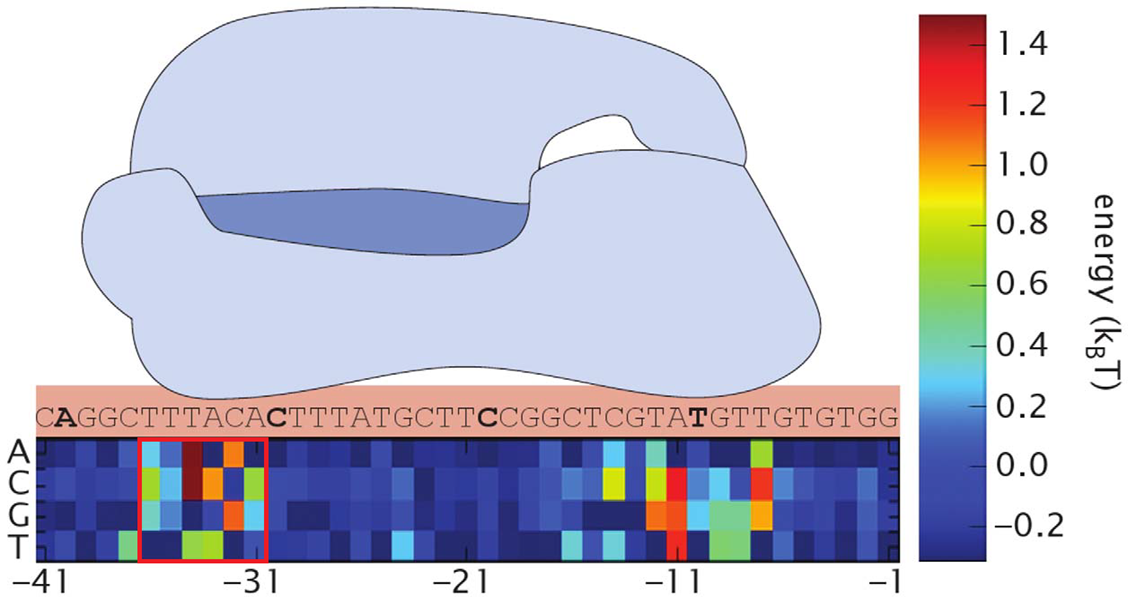fig. 1 Visualized data. A portion enclosed with red square is randomized -35 region.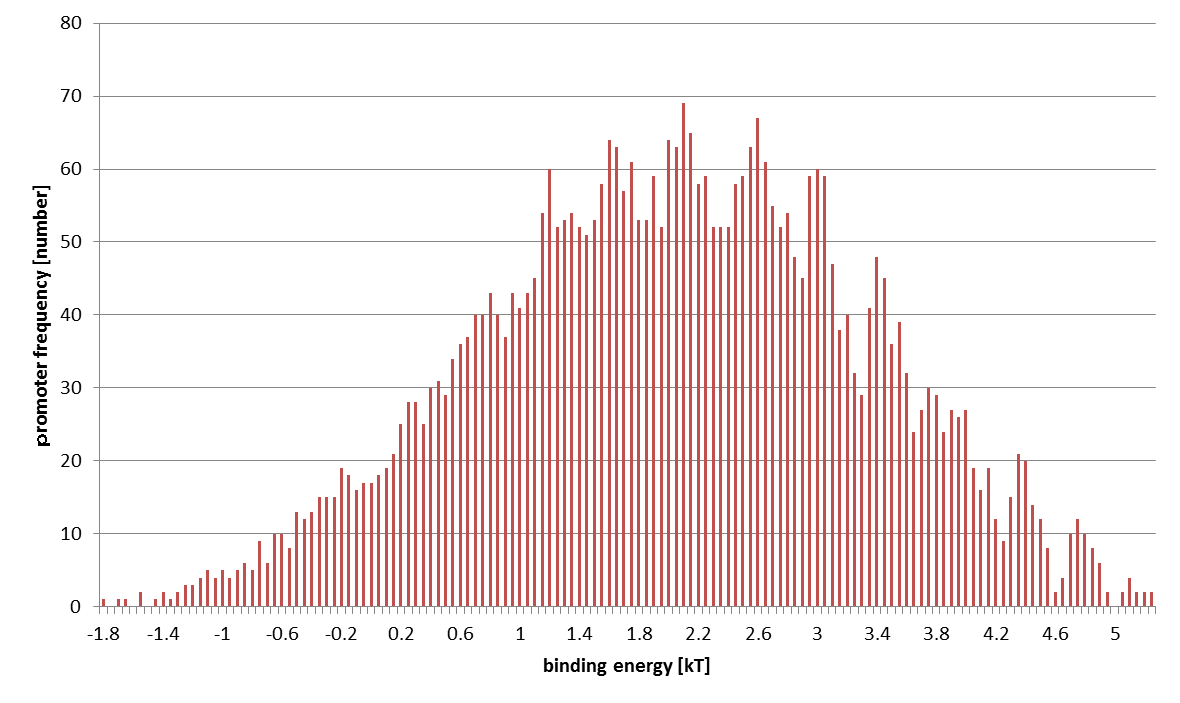fig. 2 Promoters distribution. The result is an approximate normal distribution.

## STEP 2: Conversion from Binding Energy to Binding Probability

Next, we estimated the binding probability. On this step, we used the method of statistical mechanics. So we assumed the following.

• The cell is a closed system
• There are P RNAPs bound somewhere on DNA
• The number of bases is N (bp) and 1 of N bases is +1 position of the promoter

The principle of statistical mechanics is very easy; any state emerges with the same probability. So we counted up the number of state. A state stands for any information of all the particles in the system, so the number is enormous. W represents this number. Here W can be separated as the following. $W=W_{\mathrm{unbound}}+W_{\mathrm{bound}}$ $W_{\mathrm{bound}}$ represents the number of state where the promoter is occupied and $W_{\mathrm{unbound}}$ unoccupied.

The purpose of this step is to find the ratio $W_{\mathrm{unbound}}:W_{\mathrm{bound}}$. Concerning the position of RNAP, $W_{\mathrm{unbound}}:W_{\mathrm{unbound}}&=&{\frac{N-1!}{P!(N-P-1)!} \times W_{\mathrm{R}}(E)}$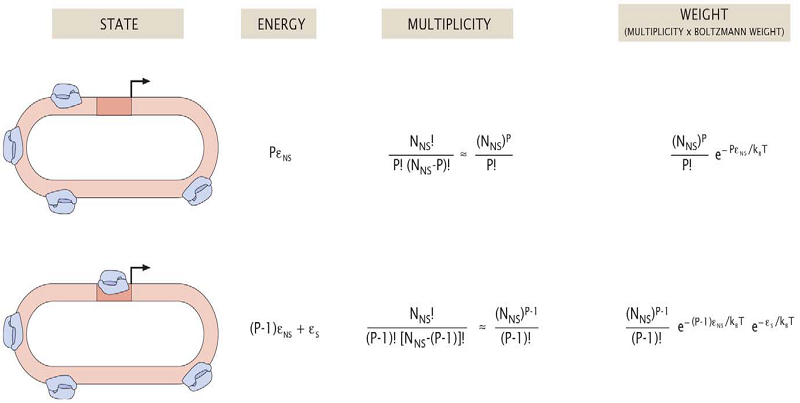M-Fig. 3 Quoted from .

Therefore, the binding probability is

\begin{align*} p&=\frac{W_{\mathrm{bound}}}{W_{\mathrm{unbound}}+W_{\mathrm{bound}}} \\[6pt] &=\frac{ \frac{P}{N_{\mathrm{NS}}} \exp\left(-\frac{\varepsilon_{\mathrm{S}} - \varepsilon_{\mathrm{NS}}}{k_{\mathrm{B}}T} \right) }{1+\frac{P}{N_{\mathrm{NS}}} \exp\left(-\frac{\varepsilon_{\mathrm{S}} - \varepsilon_{\mathrm{NS}}}{k_{\mathrm{B}}T} \right) } \\[6pt] \mathrm{suppose\ that} &\frac{P}{N_{\mathrm{NS}}} \exp\left(-\frac{\varepsilon_{\mathrm{S}} - \varepsilon_{\mathrm{NS}}}{k_{\mathrm{B}}T} \right) \ll 1 \\[6pt] &\approx \frac{P}{N_{\mathrm{NS}}} \exp\left(-\frac{\varepsilon_{\mathrm{S}} - \varepsilon_{\mathrm{NS}}}{k_{\mathrm{B}}T} \right) \\[6pt] &\propto \exp\left(-\frac{\varepsilon_{-35}}{k_{\mathrm{B}}T} \right) \end{align*}

The binding energy of -35 region is exponentially proportional to the binding probability.

The last step is to convert the binding probability to the transcription efficiency. Let us assume these suppositions.

• RNAP bound to promoter promptly initiate transcription
• There is no "traffic jam" of RNAPs on DNA (i. e., RNAP's transcription initiation is rate-limiting)

These assumptions mean that we can directly use the value of binding probability as transcription energy in an arbitrary unit. In this way, we get following conclusive result.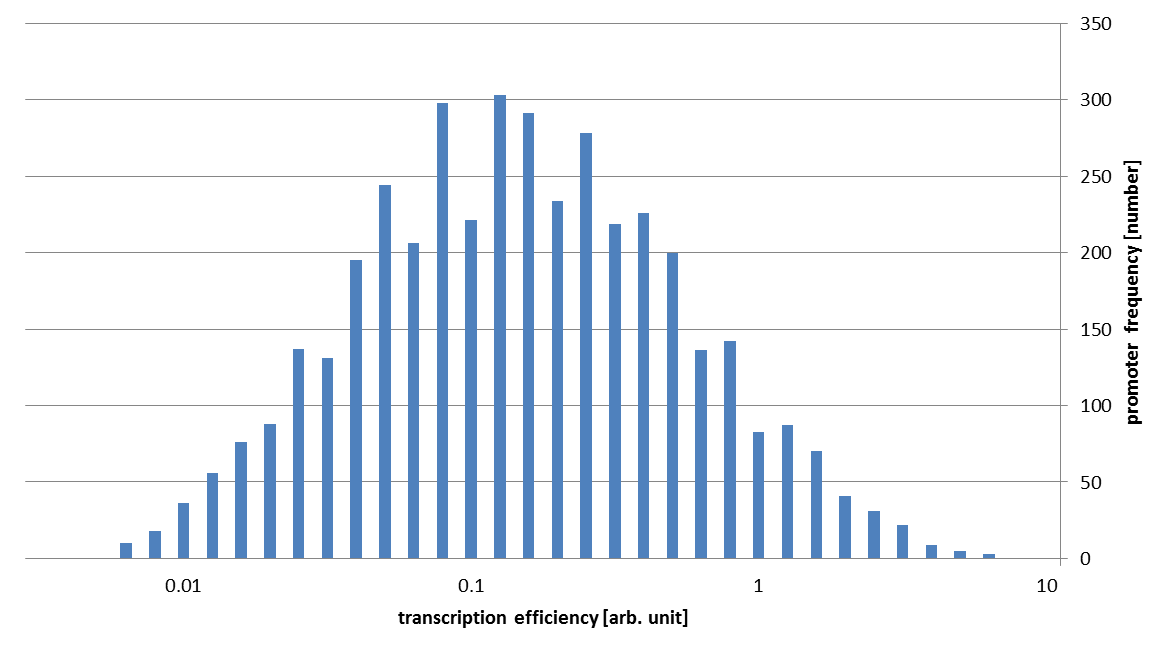M-Fig. 4 The horizontal axis stands for the transcription efficiency.

As you can see in this figure, the strengths of our promoter families vary about 1000 fold!